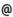## Babes-Bolyai University of Cluj-Napoca Faculty of Mathematics and Computer Science Study Cycle: Graduate SUBJECT

 Code Subject MMM0001 Mechanics
 Section Semester Hours: C+S+L Category Type Mathematics 4 2+1+1 speciality compulsory Mathematics and Computer Science 4 2+1+1 speciality compulsory Applied Mathematics 4 2+1+1 speciality compulsory
 Teaching Staff in Charge
 Prof. KOHR Mirela, Ph.D.,  mkohrmath.ubbcluj.roAssoc.Prof. SZENKOVITS Ferenc, Ph.D.,  fszenkomath.ubbcluj.roAssoc.Prof. BLAGA Cristina Olivia, Ph.D.,  cpblagamath.ubbcluj.ro
 Aims Teaching of fundamental notions of mechanics: cinematics of the material point and of rigid body, fundamental notions from the dynamics of the material point and of the rigid body. Application of the theory of differential and integral calculus theory and also of the theory of differential equations in the study of some special problems of mechanics.
 Content I. KINEMATICS: 1. Introduction. Fundamental notions. 2. Kinematics of the material point: path, motion equations, the velocity and acceleration of material point. Kinematics in Cartesian coordinates, with respect to the Frenet orthogonal axes, and in curvilinear coordinates. Areolar velocity. 3. Kinematics of the solid rigid body: Euler's angles. Motion's equations. Poisson's formulas. The velocity and accceleration distributions of solid body. The motion of the solid body with a fixed point. The general rigid-body motion. The plane-parallel motion of the rigid body. 4. The relative motion of the material point. The velocity and acceleration distributins. Coriolis' theorem. II. DYNAMICS OF MATERIAL POINTS: 1. The free material point. Principles of Newtonian mechanics. The Newton equation. Equations and general theorems. Virtual work and the force function. Central forces. Newton's problem. 2. The motion of a material point with restrictions: the motion on a fixed curve and on a fixed surface (with or without friction). The mathematical pendulum. 3. Dynamics of the relative motion: the differential equation of the relative motion. III. DYNAMICS OF SYSTEMS AND RIGID BODIES 1. Centre of inertia (gravity). Momentum of inertia. Momentum of inertia with respect to parallel axes and axes which contain a given point. Ellipsoid of inertia (principal axes and directions). Equations and general theorems for the systems of material points. Virtual work of the exterior and inner forces. Prime integrals. The motion of a material system with respect to the centre of inertia. Konig's theorems. Equations and general theorems for the motion about the centre of inertia. 2. Dynamics of the rigid body. The motion of the rigid body with a fixed point. Kinematical energy and the kinematical torque. Applications to the Lagrange-Poisson case of motion.
 References 1. ARNOLD, VLADIMIR I.: Mathematical Methods of Classical Mechanics. Berlin: Springer, 1997. 2. BRADEANU, PETRE: Mecanica Teoretica, vol. 1-2. Cluj-Napoca: Litografia Univ. Babes- Bolyai, 1988. 3. COOPER, RICHARD K. - PELLEGRINI, CLAUDIO: Modern Analytical Mechanics. New York: Kluwer Academic/Plenum Publishers, 1999. 4. DRAGOS, LAZAR: Principiile Mecanicii Analitice. Bucuresti: Ed. Tehnica, 1976. 5. GOLDSTEIN, HERBERT: Classical Mechanics. Reading, MA: Addison-Wessley (2nd ed.), 1980. 6. IACOB, CAIUS: Mecanica Teoretica. Bucuresti: Editura Didactica si Pedagogica, 1980. 7. KITTEL C., KNIGHT W.D., Cursul de Fizica Berkeley, vol. 1: Mecanica, Editura Didactica si Pedagogica, Bucuresti, 1981. 8. KOHR, MIRELA: Capitole Speciale de Mecanica, Presa Universitara Clujeana, Cluj-Napca, 2005. 9. TOROK, JOSEF. S.: Analytical Mechanics with an Introduction to Dynamical Systems. New York: John Wiley & Sons, Inc., 2000. 10. TURCU, AUREL: Mecanica Teoretica, p. I,II. Cluj-Napoca: Litografia Univ. Babes- Bolyai, 1972, 1976. 11. TURCU, AUREL - KOHR-ILE, MIRELA: Culegere de Probleme de Mecanica Teoretica. Cluj-Napoca: Litografia Univ. Babes-Bolyai, Cluj-Napoca, 1993. 12.TAYLOR, JOHN: Classical Mechanics, Ney York: Palgrave Macmillan, 2004. 13. BUDÓ Ágoston: Mechanika. Tankönyvkiadó, Budapest, 1972. 14. NAGY Károly: Elméleti mechanika. Nemzeti Tankönyvkiadó, Budapest, 1993. 15. BRADEANU, P. - POP, I. - BRADEANU, D.: Probleme si exercitii de mecanica teoretica. Ed. Tehnica, Bucuresti, 1979. 16. BRADEANU, P. et alii: Culegere de probleme de mecanica teoretica. Lit. Univ. Cluj, 1976. 17. VÎLCOVICI, V. et alii: Mecanica teoretica. Ed. Tehnica, Bucuresti, 1963. 18. BALAN, St.: Culegere de probleme de mecanica. Ed. Didactica si Pedabogica, Bucuresti, 1972.
 Assessment Exam (70%) + student activity (15%) + test paper (15%).
 Links: Syllabus for all subjects Romanian version for this subject Rtf format for this subject